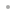Experimental Data AnalystExperimental Data AnalystFeaturesSample ImagesTable of ContentsManual Installation InstructionsBuy Online1. Introduction to Experimental Data Analyst (EDA)

• Summary and Use of EDA

• Summary of the Chapters of EDA

• Using EDA

• The EDA Data Format

• EDA Utilities and Supplied Data Sets

• Contents of the EDA `Data` Directory

2. Importing and Exporting Data

• Importing Data from an ASCII File

• Introduction

• A Real-World Example

• Summary of ImportData

• Exporting Data to an ASCII File

• Introduction

• Summary of ExportData

• Importing Data from a Binary File

• Getting Data from a Scanned Plot

3. Experimental Errors and Error Analysis

• Introduction
• The Purpose of Error Analysis

• Different Types of Errors

• References

• Determining the Precision

• The Standard Deviation

• The Error

• Rejection of Measurements

• Propagation of Errors of Precision

• Discussion and Examples

• Finding the Error in an Average

• Calibration, Accuracy, and Systematic Errors

• Summary of the Error Propagation Routines

4. Fitting Data to Linear Models by Least-Squares Techniques

• Background Discussion

• Linear Fits

• Least-Squares Techniques

• Fitting to Data with Experimental Errors

• Evaluating the Goodness of a Fit

• References

• Curve Fitting When the Data Have No Explicit Errors

• Unwinding the Residual Calculation

• Curve Fitting When the Data Have Explicit Errors

• Options, Utilities, and Details

• Options to LinearFit

• Other Routines in the LinearFit Package

• Summary of the LinearFit Package

• The LinearFit Routine

• The ShowLinearFit Routine

• The ToLinearFunction Routine

5. Fitting Data to Nonlinear Models

• Introduction

• Overview of FindFit

• Providing Initial Parameter Values to FindFit

• Comparing LinearFit and FindFit

• References

• Examples

• Fitting to a Single Peak with a Background

• Fitting to Two Peaks with No Background

• Options, Utilities, and Details

• Options to FindFit

• Other Routines in the FindFit Package

• Peak-Shape Routines

• Summary of the FindFit Package

6. Smoothing Data, Filling Missing Data, and Nonparametric Fitting

• Introduction

• Smoothing with Averaging Techniques

• Fourier Filters

• Loess Fitting

• Using Mathematica Built-In Functions

• Smoothing an Interpolation

• Filling Missing Data

• References

• Details

• Nonmonotonic Data and Related Assumptions

• Comparing Various Smoothing Methods

• How LoessFit Works

• Summary of the SmoothData Package

7. Robust Fitting

• Introduction

• Using the Median

• Using Weighting Techniques

• Details

• RobustCurveFit

• RobustLineFit

• Comparing RobustCurveFit and RobustLineFit

• Summary of the RobustFit Package

8. Exploration and Transformation of Data

• Graphical Exploration

• EDAListPlot

• Histogram

• BoxPlot

• Summary of General Purpose EDA Graphics Routines

• Transforming Data

• The Data Itself Suggests a Transformation

• Transforming to Match a Model

• Finding Hidden Features of the Data

• Estimating Parameters for Peaks

• Summary of FindPeaks

• FitPeaks and FitExponent

• FitPeaks

• FitExponent

• References

• References to Mathematica

• References to Exploratory Data Analysis and Transformation